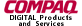gluDisk.3glOpenGL man pages

```

```

## Name

```  gluDisk - draw a disk

```

## C Specification

```  void gluDisk(	GLUquadricObj *qobj,
GLint slices,
GLint loops )

```

## Parameters

```

slices       Specifies the number of subdivisions around the z axis.

loops	       Specifies the number of concentric rings	about the origin into
which the disk is subdivided.

```

## Description

```  gluDisk renders a disk on the	z = 0  plane.  The disk	has a radius of
innerRadius.	If innerRadius is 0, then no hole is generated.	 The disk is
subdivided around the	z axis into slices (like pizza slices),	and also
about	the z axis into	rings (as specified by slices and loops,
respectively).

With respect to orientation, the +z side of the disk is considered to	be
"outside" (see gluQuadricOrientation).  This means that if the orientation
is set to GLU_OUTSIDE, then any normals generated point along	the +z axis.
Otherwise, they point	along the -z axis.

If texturing is turned on (with gluQuadricTexture), texture coordinates are
generated linearly such that where r=outerRadius, the	value at (r, 0,	0) is
(1, 0.5), at (0, r, 0) it is (0.5, 1), at (-r, 0, 0) it is (0, 0.5), and at
(0, -r, 0) it	is (0.5, 0).

```

```  gluCylinder, gluNewQuadric, gluPartialDisk, gluQuadricOrientation,